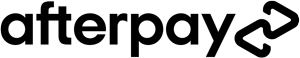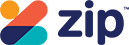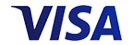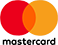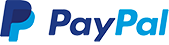\$49.95
Increase value Decrease value
\$49.95
Increase value Decrease value
WAS \$59.95 NOW \$29.98
Increase value Decrease value
WAS \$44.95 NOW \$22.47
Increase value Decrease value
\$49.95
Increase value Decrease value
\$29.95
Increase value Decrease value
\$415.00
Increase value Decrease value
\$150.00
Increase value Decrease value
\$195.00
Increase value Decrease value
\$485.00
Increase value Decrease value
\$435.00
Increase value Decrease value
\$219.00
Increase value Decrease value